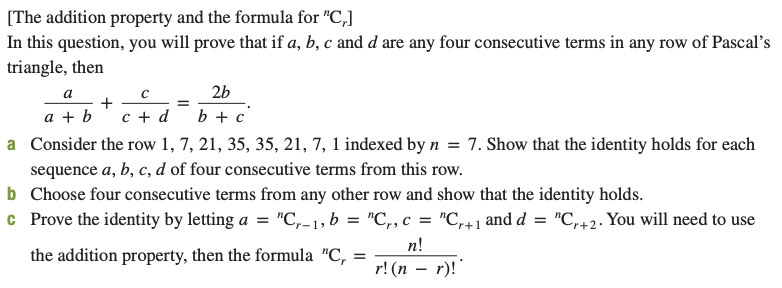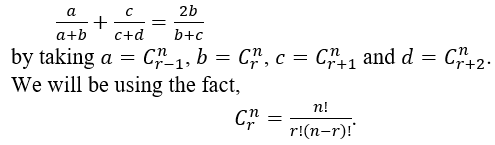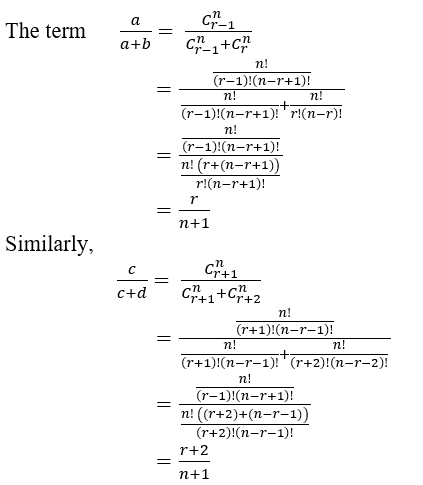# [The addition property and the formula for "C,]In this question, you will prove that if a, b, c and d are any four consecutive terms in any row of Pascal'striangle, then2bb + cc + da + ba Consider the row 1, 7, 21, 35, 35, 21, 7, 1 indexed by n = 7. Show that the identity holds for eachsequence a, b, c, d of four consecutive terms from this row.b Choose four consecutive terms from any other row and show that the identity holds.c Prove the identity by letting a ="C,-1, b = "C,, c = "C,+1 and d = "C,+2. You will need to usen!r! (п — г)!the addition property, then the formula "C,

Question
1 views

chelp_outlineImage Transcriptionclose[The addition property and the formula for "C,] In this question, you will prove that if a, b, c and d are any four consecutive terms in any row of Pascal's triangle, then 2b b + c c + d a + b a Consider the row 1, 7, 21, 35, 35, 21, 7, 1 indexed by n = 7. Show that the identity holds for each sequence a, b, c, d of four consecutive terms from this row. b Choose four consecutive terms from any other row and show that the identity holds. c Prove the identity by letting a = "C,-1, b = "C,, c = "C,+1 and d = "C,+2. You will need to use n! r! (п — г)! the addition property, then the formula "C, fullscreen
check_circle

Step 1

We need to show the identity,Step 2

So,...

### Want to see the full answer?

See Solution

#### Want to see this answer and more?

Solutions are written by subject experts who are available 24/7. Questions are typically answered within 1 hour.*

See Solution
*Response times may vary by subject and question.
Tagged in

### Math